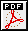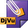# Dimitry Leites - Workouts of SoS, 1977-2003

[Books and chapters in books] [Books edited] [Books translated] [Papers] [Encyclopedia entries] [Workouts of SoS]

Here is the list of workouts of Seminar on Supermanifolds (later renamed, more appropriately, Seminar on Supersymmetries) or SoS during 1977-2003 and preprinted in Reports of the Department of Mathematics, Stockholm University, Sweden. The number N/year-n denotes SoS-preprint N published in year indicated as n-th report of the Department. Titles of several preprints differ from those of published vershions; we provide with the published one.

Some results have, at least apparently, nothing to do with supersymmetry and were preprinted here at the pre-glasnost time, when some Soviet authors found it difficult or impossible to get an official permission to publish their paper but were willing to take a risk and preprint their result "illegally" but in English. Label s marking a contribution means "to be superized".

## 1/1986-21

D. Leites, Selected problems of supermanifold theory, 1-21.
• Published in .

T. Khovanova, Lie superalgebra structure on eigenfunctions, and jets of the resolvent's kernel near the diagonal of an $$n$$th order ordinary differential operator, 22-39.
A. Radul, Superstring Schwarz derivative and the Bott cocycle, 48-57.

T. Khovanova, Korteweg-de Vries superequation related to the Lie superalgebra of Neveu-Schwarz-2 string theory, 58-73.

s V. Sokolov, Finite-dimensional subalgebras in $$K(3|0)$$ and evolutionary equations, 74-89.

## 2/1986-22

s J. Bernstein and O. Schwarzman, Complex crystallographic Coxeter groups and affine root systems; id., Chevalley's theorem for the complex crystallographic groups, 66 pp.

## 3/1986-23

s J. Bernstein and S. Gindikin, Four papers on integral geometry and Einstein equations, 61 pp.

## 4/1986-24

V. Drinfeld, Quantum groups, 1-32.

D. Gurevich, Quantum Yang-Baxter equation and a generalization of the formal Lie theory, 33-123.
For a bit shorter version, see:
• The Yang-Baxter equation and the generalization of formal Lie theory, Dokl. Akad. Nauk SSSR 288 (1986), no.4, 797-801 (in Russian); English translation: Soviet Math. Doklady 33 (1986), no.3, 758-762. MR 88b:58066

## 5/1986-25

s B. Feigin and D. Fuchs, Representations of Virasoro algebra, 70 pp.

## 6/1987

M. Antonets and A. Tsvetkov, Algebras of random variables and their representations, 27 pp.
Published in:
• Selecta Math. Soviet. 8 (1989), no.3, 271-289. MR 91a:28010

## 7/1987

S. Merkulov, Superconformal invariance of $$N=1$$ supersymmetric theories, 20 pp.

## 8/1987

J. Donin, Skew diagrams and decomposition into irreducible components of the exterior and symmetric powers of the adjoint representations of $$GL(n)$$ and $$GL(p|q)$$, 1-38.
Partly published in:
• Decompositions of tensor products of representations of a symmetric group and of symmetric and exterior powers of the adjoint representation of $$\mathfrak{gl}(N)$$, Dokl. Akad. Nauk SSSR 303 (1988), no.6, 1296-1301 (in Russian); English translation: Soviet Math. Dokl. 38 (1989), no.3, 654-658. MR 91b:17007
• Decomposition of symmetric and exterior powers of the coadjoint representation of $$\mathfrak{gl}(n)$$ in the unstable case, Funkts. Anal. Prilozh. 26 (1992), no.4, 70-73 (in Russian); English translation: Funct. Anal. Appl. 26 (1992), no.4, 287-289. MR 94c:17014

s P. Grozman, On bilinear covariant differential operators acting on the space of tensor fields on a symplectic manifold, 39-44.
• Published in [G].

A. Onishchik, The action of Lie superalgebras of Cartan type on some split supermanifolds, 45-53.
Published in:
• Actions of Cartan-like Lie superalgebras on some supermanifolds, Problems in group theory and in homological algebra, Yaroslavl State Univ., 1989, 42-49 (in Russian). MR 91k:58007

## 9/1987-13

J. Bernstein, Two papers for pedestrians on representations of Lie algebras and Lie superalgebras, 60 pp.
• See [E5] and [E8], respectively.

## 10/1987-14

s J. Bernstein and S. Gelfand, $$\Lambda(\Theta)$$-modules and algebraic vector bundles on $$\mathbb P(\Theta)$$, 28 pp.Partly published in:
• S.I. Gelfand, Sheaves on $$\mathbb P^n$$ and problems in linear algebra, Appendix in: C. Okonek, M. Schneider and H. Spindler, Vector bundles on complex projective spaces, translated from the English by V.Ya. Lin; translation edited and with a preface by Yu.I. Manin, Mir, Moscow, 1984 (in Russian). MR 86i:14005## 11/1987-15

Two papers on differential geometry:

J. Bernstein, Admissible complexes of curves, 1-15.

s A. Goncharov, Generalized conformal structures on manifolds, 16-70.

## 12/1987-16

O. Ogievetsky, Two papers on $$K_2$$-functors for superalgebras, 30 pp.

## 13/1987-17

G. Ilyuta, On monodromy groups and Dynkin diagrams, 23 pp. The result bridges singularities and root systems over $$\mathbb Z/2$$; to understand explicit relation with superroot systems is an open problem.

## 14/1987-18

M. Finkelberg, Graded algebras and Brauer groups, 30 pp.

## 15/1987-19

V. Lyubashenko, Vectorsymmetries, 77 pp.

## 16/1987-20

I. Gelfand and Yu. Daletskii, Lie superalgebras and Hamilton operators, 26 pp.
Partly published in:
• I.M. Gelfand, Yu.L. Daletskii and B.L. Tsygan, On a variant of noncommutative differential geometry, Dokl. Akad. Nauk SSSR 308 (1989), no.6, 1293-1297 (in Russian), English translation: Soviet Math. Dokl. 40 (1990), no.2, 422-426. MR 91j:58015
• Yu.L. Daletskii, Lie superalgebras in a Hamiltonian operator theory, Nonlinear and turbulent processes in physics, Vol. 3, Harwood Academic Publ., Chur, 1984, 1289-1295. MR 87e:58066

## 17/1987-23

I. Volichenko, Nonhomogeneous subalgebras of commutative superalgebras, 17 pp.
• Published as appendix in .

## 18/1987-24

V. Ginzburg, Twisted cotangent bundles and twisted differential operators, 11 pp.

## 19/1987-25

F. Weinstein, Cohomology of braid groups, 1-20.

s B. Feigin, On cohomology of the Lie algebra of vector fields and of the current algebra, 21-50.
Published in:
• Selecta Math. Soviet. 7 (1988), no.1, 49-62. MR 90c:17026

## 20/1987-26

A. Onishchik, Transitive Lie superalgebras of vector fields, 1-21.

A. Serov, Vector fields on split supermanifolds, 22-81.
Partly published in:
• A.L. Onishchik and A.A. Serov, Lie superalgebras of vector fields on splittable flag supermanifolds, Dokl. Akad. Nauk SSSR 300 (1988), no.2, 284-287 (in Russian); English translation: Soviet Math. Dokl. 37 (1988), no.3, 652-655. MR 89i:32067

## 21/1987-26

V. Serganova and A. Vaintrob, Simple singularities of functions on supermanifolds, 59 pp.

## 22/1988-4

A. Sergeev, Irreducible representations of solvable Lie superalgebras, 1-12.

V. Serganova, Automorphisms and real structures of Lie superalgebras, 13-99.

V. Serganova, Super Weyl groups and dominant weights, 100-132.
Used in:
• G. Egorov, How to superize $$\mathfrak{gl}(\infty)$$, Topological and geometrical methods in field theory (ed. J. Mickelsson and O. Pekonen), World Scientific, 1992, 135-146. MR 94c:17007

## 23/1988-5

Several papers on equations of mathematical physics, 76 pp.:

D. Gurevich, Appendices to 4/1986. Hecke symmetries and quantum determinants, 1-16.

D. Leites, Corrections to , 17-18.

D. Leites and E. Poletaeva, Two remarks on Lie superalgebras of string theories, 19-22.
• Used in .

s V. Ovsienko and O. Udalova, Projective structures and infinite-dimensional Lie algebras associated with a contact manifold, 23-59.

T. Khovanova and O. Udalova, Superversions of Miura transformations, 60-66.

T. Khovanova, Sturm-Liouville operators connected with superanalogs of Virasoro algebra, 67-76.
• The last two papers see in [B7].

## 24/1988-6

A. Vaintrob, Deformations of complex structures on supervarieties, 144 pp.

## 25/1988-7

s A. Rosenberg, Almost quotient categories, sheaves and localization, 181 pp.

## 26/1988-8

A. Rosenberg, Noncommutative affine semischemes and schemes, 317 pp.
Published in:
• Noncommutative algebraic geometry and representations of quantized algebras, Mathematics and its Applications, 330. Kluwer, 1995. xii+315 pp. MR 97b:14004
• Preprints MPIM-Bonn 1999-83, 1999-84, 2003-110, 2003-111, 2003-112.

## 27/1988-9

s I. Miklashevsky, Connections, conformal structures and Einstein equations, 31 pp.

## 28/1988-10

s B. Bloch, Instantons and superpotential for string theory on K3-surfaces, 11-16.

s V. Lychagin, V. Rubtsov and I. Chekalov, A classification of Monge-Ampère equations, 17-69.

## 29/1988-11

A. Tolpygo, Lie algebra cohomology, its weights and generating functions, 34 pp.

## 30/1988-13

Textbook on supermanifolds and Lie superalgebras.
Introduction (after Manin's [E6]), 1-23.

Chapter 0. Lectures on algebraic geometry (by/after Manin's [E6]), 24-157.

J. Bernstein, D. Leites and V. Shander, Algebra and calculus on supermanifolds, 158-313.

## 31/1988-14

Continuation of 30/1988 with addenda to 22/1988, 25/1988, 26/1988.

J. Bernstein and D. Leites, Algebra and calculus on supermanifolds, Chapters 3-5, 1-44, 135-172.

V. Shander, Integration theory on supermanifolds, 45-131.

D. Leites and I. Shchepochkina, On classification of simple infinite dimensional Lie superalgebras of vector fields, 235-278.

D. Leites, J.P. Serre-I.L. Kantor relations of the classical Lie superalgebras, 279-289.
• Published in  and .

## 32/1988-15

I. Shchepochkina, Maximal subalgebras of classical Lie superalgebras, 1-43.

A. Sergeev, Invariant functions and Laplace-Casimir operators on Lie superalgebras, 44-95.
• Published in [S1], [S2] and .

## 33/1989-2

F. Weinstein, On birational automorphisms of Severi-Brauer surfaces, 14 pp.

## 34/1989-11

s D. Gurevich, Algebraic aspects of the quantum Yang-Baxter equation, 1-47.

V. Serganova, Simple Volichenko algebras, 48-52.

## 35/1990-9

s M. Zhitomirskii, Typical singularities of differential $$1$$-forms and Pfaffian equations, 1-285.

D. Leites and E. Poletaeva, Analogues of the Riemannian structure on supermanifolds, 286-296.
• Published in .

E. Poletaeva, Structure functions on $$(2|2)$$-dimensional supermanifolds with either of the differential forms $$d\xi^{\frac{2\lambda -1}{1-\lambda}}((1-\lambda )dpdq + \lambda d\eta d\xi)$$ or $$d\xi^{\frac{\lambda}{\lambda -2}}(dpdq + d\eta d\xi)$$, where $$\lambda \in \mathbb C$$, 297-304.

## 36/2003

E. Poletaeva, The analogs of Riemannian and Penrose tensor on supermanifolds, Preprint MPIM-Bonn 2003-19; arXiv:math/0510165, 71 pp.

## 37/2003

D. Leites and P. Grozman, SuperLie and problems (to be) solved with it, Preprint MPIM-Bonn 2003-39, 61 pp.

Somewhat belatedly we have decided
let us save trees and put preprints in arXiv only.